# Fast Target Recognition Method Based on Multi-Scale Fusion and Deep Learning

Fast Target Recognition Method Based on Multi-Scale Fusion and Deep Learning
Department of Electrical and Information Engineering, Hebei Jiaotong College of Vocational and Technical, Shijiazhuang 050051, China

Road Traffic Perception and Intelligent Application Technology R&D Center of Universities in Hebei Province, Shijiazhuang 050051, China

Corresponding Author Email:
kuangbo@hejtxy.edu.cn
Page:
2173-2179
|
DOI:
https://doi.org/10.18280/ts.390631
15 August 2022
|
Accepted:
20 November 2022
|
Published:
31 December 2022
| Citation

OPEN ACCESS

Abstract:

Under the natural state of unrestricted conditions, the accuracy of effective recognition of image target information captured by ordinary cameras is significantly reduced. At present, the mainstream research methods for image target recognition focus on the processing of images based on algorithms with strong ability to describe image target features so as to improve the image target recognition performance in cases of complex noise interference. However, most of the methods cannot adapt to various changes in the background when the environment changes. Therefore, this article conducts studies on the fast target recognition method based on multi-scale fusion and deep learning. The optimized local binary pattern algorithm and the HOG algorithm are used to extract the image target features, the dimension reduction of the extracted image target features is carried out based on the generalized discriminant analysis algorithm, and multi-scale fusion of image targets is accomplished based on the discriminant correlation analysis. The experimental results verify the effectiveness of the proposed algorithm.

Keywords:

multi-scale fusion, deep learning, fast target recognition

1. Introduction

There are many application scenarios of environment perception technology based on image processing, and it is of great practical significance to carry out relevant researches [1-5]. Along with the diversification of shooting scenes, the demand for environment perception such as target recognition and tracking in images is increasing [6-11]. However, in indoor scenes shaded with obstacles or outdoor open scenes, the accuracy and speed of existing image processing technologies for target recognition cannot yet meet the actual demand, and the accomplishment of environment perception tasks is far from satisfying [12-17]. In reality, many image target recognition software and hardware products have been put into the market. However, under the natural state of unrestricted conditions, the accuracy of effective recognition of image target information captured by ordinary cameras is greatly reduced [18-21]. In order to improve the robustness of image target recognition in complex environments, researches on related key technologies are needed.

Image target detection and recognition has been widely used in many fields. However, the robustness of existing methods is poor with a high error rate in target recognition and high dependence on parameters, thus they are limited in application. Therefore, an image target detection and recognition method based on an improved R-CNN model is proposed in the reference  in an effort to detect and recognize dynamic image targets in real time. In order to improve the accuracy and real-time performance of the model in image target detection and recognition, a target feature matching module is used in the existing R-CNN network model and a feature map close to the same target is obtained by calculating the similarity of the features extracted from the model. In view of low accuracy of small target recognition, a small target recognition method based on improved Faster_Rcnn is proposed in reference . As the ROI pool method in Faster Rcnn may lead to quantization error in operation, inaccurate positioning and other problems in detection, an improved ROI alignment method is adopted to eliminate quantization error. In order to deal with the problem of target recognition in synthetic aperture radar (SAR) images, a multi-view method is proposed in reference . For the multi-viewpoint SAR images to be recognized, they are first clustered based on correlation coefficients and divided into multiple viewpoint sets. Then the view sets containing two or more images are fused into a single feature vector using multi-set canonical correlation analysis (MCCA). Convolution neural network has achieved excellent performance in a wide range of applications, but its huge resource consumption poses a great challenge to its application in mobile terminals and embedded devices. To solve these problems, it is necessary to balance the size, speed and accuracy of the network model. A new shallow neural network based on ResNet and DenseNet is proposed in the reference . Convolutional kernels of different sizes are used to obtain feature maps, which are then concaved. Two convolutional layers are then constructed to reduce the size of the feature map and increase the depth of the network. To construct a CT-based automatic target identification screening system for airports, a method combining two popular techniques, CT image processing and machine learning, is introduced in the reference . Using grayscale features and Histogram of Oriented Gradients (HOG) features extracted from CT images, different classifiers (SVM, KNN) can be trained to identify desired targets (brine, rubber, clay). By comparing the recall rate and precision of each classifier, the best classifier for this project can be found.

Based on the existing research results, it can be found that in order to improve the performance of image target recognition in cases of complex noise interference, the current mainstream research method for image target recognition is to process images based on algorithms with strong ability to describe image target features. Most methods focus the study on a single shooting scene or a single feature of the image target, making the model unable to adapt to multiple changes in the background as the environment changes. In order to better deal with the degradation of image target recognition performance in complex environments, this article investigates the fast target recognition method based on multi-scale fusion and deep learning. In the second chapter, the optimized local binary pattern algorithm and HOG algorithm are used to extract the image target features. In the third chapter, the dimension of the extracted image target features is reduced based on the generalized discriminant analysis algorithm. In the fourth chapter, the multi-scale fusion of image target is accomplished based on the discriminant correlation analysis method. The experimental results verify the effectiveness of the proposed algorithms.

2. Feature Extraction Method for Fast Target Recognition

# 1.png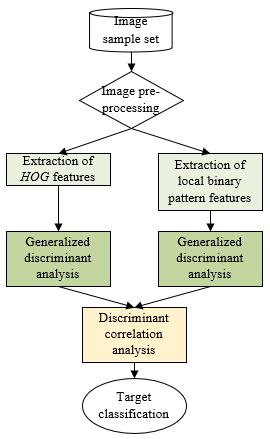In the traditional artificial intelligence field, to achieve fast recognition of targets in images, it is usually necessary to first extract the target features, followed by dimension reduction of features, and finally the recognition and classification of the targets implemented through classification algorithms. However, the recognition performance of algorithms with similar target recognition ideas is susceptible to interference from many factors such as illumination changes, shading by obstacles, poses as well as expressions. To tackle this problem, one of the more effective approaches is to extract the illumination invariant features of the target in the image. Normally, the target image to be recognized includes some feature information that is insensitive to illumination changes such as contour gradient information or local feature information. If such feature information can be extracted, the accuracy of fast target recognition of models in complex environments will be greatly improved.

In order to achieve the complementary advantages between different image target features, this article first extracts the image target features, and then performs dimension reduction and fusion over the obtained image target features. As for selected feature extraction algorithms, the optimized local binary pattern algorithm and the HOG algorithm are used. As for the feature dimension reduction and fusion algorithms, the generalized discriminant analysis and discriminant correlation analysis methods are adopted. The flow chart of the algorithm in this article is shown in Figure 1.

## 2.png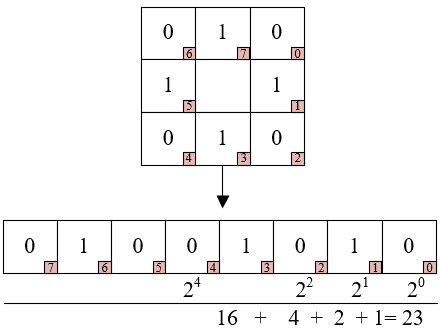Figure 2. Example of local binary pattern feature calculation

The local binary pattern algorithm has the advantages in rotation invariance as well as gray scale invariance and is negligibly affected by illumination. The basic principle of this algorithm is to compare the gray value of the central pixel of the image with that of its neighboring pixels, and to arrange the calculated local binary pattern features in clockwise order, and the decimal value obtained by the weighting operation is the final local binary pattern feature. Figure 2 shows an example of local binary pattern feature calculation. Assuming that the pixel values of m neighborhoods centered on hd are represented by hi(i=1, 2,..., m), the radius of the local binary pattern descriptor is represented by s, the Boolean functional expression is as follows:

LB{{P}_{M,S}}=\sum\limits_{i=0}^{M-1}{r\left( {{h}_{i}}-{{h}_{d}} \right){{2}^{i}}},r\left( a \right)=\left\{ \begin{align} & 1,a\ge 0 \\ & 0,otherwise \\\end{align} \right.      (1)

In the existing local binary pattern algorithms, the middle pixel value is selected as the central reference threshold, but if the value is too high, all other values in the neighborhood will become 0 or 1, making the overall image too bright or too dark so that the filtering illumination processing is invalid. In order to solve the above problems, this article modifies the calculation method for benchmark threshold of the algorithms and the measurement formula of local binary pattern features, then extracts multi-scale features of targets in image samples, and fully explores the global and local features that contain more image target texture information while avoiding the influence of noise such as illumination changes. The improved algorithm flow is detailed as follows:

Step 1: Assuming that the number of pixel blocks in the neighborhood is represented by m, the central threshold of the pixels in the image neighborhood can be calculated based on the following formula:

${{t}_{c}}=\frac{\sum\limits_{i=1}^{m}{{{t}_{i}}}}{m}$     (2)

Step 2: Calculate the absolute value of the difference between the pixel value and the central value in the image neighborhood, and the result is compared with the standard deviation of the pixel values in the neighborhood. The formula for calculating the standard deviation is shown in the following formula. If the calculation result is greater than the standard deviation, it is denoted as 1 or as 0 if less than the standard deviation.

$\varepsilon =\sqrt{\frac{\sum\limits_{i=1}^{m}{{{\left( {{t}_{i}}-{{t}_{d}} \right)}^{2}}}}{m-1}}$     (3)

Step 3: Calculate the image target features extracted by the local binary pattern operator based on the following formula:

LB{{P}_{M,S}}^{*}=\sum\limits_{i=0}^{M-1}{r\left( {{t}_{i}}-{{t}_{d}} \right){{2}^{i}}},r\left( a \right)=\left\{ \begin{align} & 0,\left| a \right|\le \varepsilon \\ & 1,\left| a \right|>\varepsilon \\\end{align} \right.      (4)

Step 4: Local binary pattern features of uniform pattern can be obtained by filtering all scale features of image sample target, and combining feature histograms of multiple image samples can generate desired multi-scale local binary pattern features. Figure 3 shows an example of the feature extraction of the improved local binary pattern algorithm.

## 3.png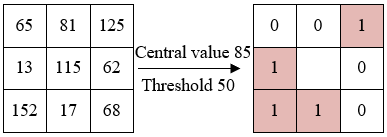Figure 3. An example of improved local binary pattern algorithm feature extraction

The HOG algorithm characterizes the image target features by calculating the local gradient direction and size of the image, and the algorithm has higher resistance to changes in illumination or image shape. The main procedure of the algorithm is detailed below:

Step 1: Pre-processing of image: graying and Gamma normalization;

$Gray=0.3\times R+0.6\times G+0.1\times B$      (5)

$B(a, b)=F(a, b)^\lambda$     (6)

## 4.png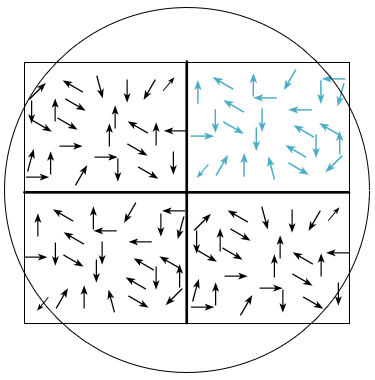Figure 4. Derivation of gradient direction

Step 2: After the above two steps of image pre-processing, use one-dimensional template vectors [-101] and [-1 0 1]T to calculate and derive the gradient value and gradient direction of each pixel value of image samples in the horizontal and vertical directions. Figure 4 shows the gradient direction derivation:

${{H}_{a}}\left( a,b \right)=F\left( a+1,b \right)-F\left( a-1,b \right)$     (7)

${{H}_{b}}\left( a,b \right)=F\left( a,b+1 \right)-F\left( a,b-1 \right)$    (8)

$H\left( a,b \right)=\sqrt{{{H}_{a}}{{\left( a,b \right)}^{2}}+{{H}_{b}}{{\left( a,b \right)}^{2}}}$      (9)

$\omega \left( a,b \right)=\arctan \left( \frac{{{H}_{b}}\left( a,b \right)}{{{H}_{a}}\left( a,b \right)} \right)$     (10)

Step 3: Based on the gradient value matrix and gradient direction matrix, each pixel point of the image sample therefore obtains its own gradient value and gradient direction. The original image sample is divided into X blocks of 16×16 size, with each block containing Y cells of 8×8 size. Finally, all the gradient values are split according to the direction to form Z bins that record the gradient values that conform to the direction angle range, and finally the HOG gradient histogram can be obtained. Figure 5 shows the splitting results of gradient value direction.

## 5.pngFigure 5. Splitting results of gradient value direction

3. Feature Dimension Reduction Method for Fast Target Recognition

In order to obtain more accurate target features in complex environments, the multi-scale local binary pattern algorithm and the HOG algorithm are modified in this article to obtain better robustness of target recognition. However, at the meantime, the dimensionality of the extracted target features appears to be too high to solve, and the redundant information contained in the feature information leads to a significant increase in the algorithm operations. In order to improve the calculation speed and target recognition performance of the algorithm, this article uses the generalized discriminant analysis algorithm for feature dimension reduction. Essentially, this algorithm uses the nonlinear kernel function as a mapper to map the input image samples to G through Hilbert space transformation. The mapping process is given by the following formula:

$\tau :A\to G,a\to \tau \left( a \right)$     (11)

The linear judgment analysis will be used in Hilbert space after the mapping is completed.

Assuming that there are M types of targets to be recognized in the image sample training set A, the i-th sample can be represented by ai=(i=1, 2, , ... N), and the number of samples in each subclass Ai(l=1, 2, . . . M, ) is represented by mk, thus the equation ∑k=1Mmk=N holds. In Hilbert space, the inter-class dispersion is represented by Ry, the intra-class dispersion by Rq, the mean of the subset of class k samples by nk, the overall mean of the samples by n, and the j-th sample of the subset of the class k samples in Hilbert space by τ(Ajk). The following formulas demonstrates the definitions of the two types of dispersion:

${{R}_{y}}=\sum\limits_{k=1}^{M}{{{m}_{k}}}\left( {{n}_{k}}-n \right){{\left( {{n}_{k}}-n \right)}^{T}}$       (12)

${{R}_{q}}=\sum\limits_{k=1}^{M}{\sum\limits_{j=1}^{{{m}_{j}}}{\left( \tau \left( A_{l}^{j} \right)-{{n}_{l}} \right){{\left( \tau \left( A_{l}^{j} \right)-{{n}_{k}} \right)}^{T}}}}$       (13)

From the definition, the following equations can be constructed for solving:

$\mu {{R}_{q}}u={{R}_{y}}u$      (14)

The eigenvectors whose eigenvalues are maximal can be represented by τ(Atw) in the above formula, so there exists xtw satisfying the following formula.

$u=\sum\limits_{t=1}^{M}{\sum\limits_{w=1}^{{{m}_{t}}}{x_{t}^{w}\tau \left( A_{t}^{w} \right)}}$      (15)

The inner product of sample subsets t and w in the nonlinear space can be calculated by the following formula.

${{\left( {{l}_{ij}} \right)}_{tw}}={{\left( \tau \left( A_{t}^{i} \right) \right)}^{T}}\tau \left( A_{w}^{j} \right)$      (16)

Meanwhile, there exists a block diagonal matrix of size N × N as follows. Let the matrix with element values of 1 /N and size Ni×Ni be represented by Qi, thus:

$Q={{\left( {{Q}_{i}} \right)}_{i=1,...,M}}$      (17)

By solving Formula 14, the following formula can be obtained:

$\mu LLx=LQLx$      (18)

The vector x can be obtained by matrix operations, and the projection matrix can be represented by V = (x1,..., xc), and after dimension reduction of the extracted target features by the generalized discriminant analysis algorithm, the features with dimension M-1 can be obtained.

$b={{l}_{a}}V$       (19)

4. Feature Fusion Method for Fast Target Recognition

The purpose of the discriminant correlation analysis algorithm is to find a set of linear projection vectors that maximize the correlation of similar features, effectively reducing the redundant information of correlation between different kinds of image samples. In this article, the correlation between features is used as the fusion decision, thus achieving the fusion of the multi-scale features of the extracted image target.

Assuming there are d image classes in the image sample set. The feature matrices of the two sets of samples in the image sample set are represented by A and B. The feature vector of the j-th sample in the i-th class of samples of A is represented by aij, the total number of samples is represented by m, and the number of samples in the i-th class is represented by mi. The formulas for calculating the average values ai* and a* of intra-class features and inter-class features are as follows,

${{a}_{i}}^{*}=\frac{1}{m}\sum\limits_{j=1}^{m}{{{a}_{ij}}}$     (20)

${{a}^{*}}=\frac{1}{m}\sum\limits_{i=1}^{d}{{{m}_{i}}{{a}_{i}}^{*}}$     (21)

The inter-class difference of different class sample features in the sample group is represented by ψya, and the inter-class scatter matrix Rya can be obtained by the following formula:

${{R}_{ya}}=\sum\limits_{i=1}^{d}{{{m}_{i}}\left( {{a}_{i}}^{*}-{{a}^{*}} \right){{\left( {{a}_{i}}^{*}-{{a}^{*}} \right)}^{T}}}={{\psi }_{ya}}\psi _{ya}^{T}$     (22)

The formula for calculating ψya is as follows:

${{\psi }_{ya}}=\left[ \sqrt{{{m}_{1}}}\left( {{a}_{1}}^{*}-a \right),\sqrt{{{m}_{2}}}\left( {{a}_{2}}^{*}-a \right),...,\sqrt{{{n}_{d}}}\left( {{a}_{d}}^{*}-a \right) \right]$     (23)

When the dimension of the eigenvector satisfies t >>d, the main eigenvector for image target recognition can be obtained by converting into (τTyaψya)d×d, where τTyaψya is a diagonal matrix. If the difference between the non-similar features of the image target is obvious, assuming that the eigenvector matrix of the orthogonal matrix is represented by W, then:

${{W}^{T}}\left( \tau _{ya}^{T}{{\psi }_{ya}} \right)W=\Omega$      (24)

Arrange the feature vectors of the image target in descending order, and form ψ(d×l) based on the first l features in the sequence, thus:

${{\left( {{\psi }_{ya}}\delta \right)}^{T}}{{R}_{ya}}\left( {{\psi }_{ya}}\delta \right)={{\Omega }_{\left( l\times l \right)}}$      (25)

Assuming that the transformation matrix is represented by Q ya=ψyaδΓ1/2, unitize Rya, and the dimension of matrix A is reduced from t to l based on the following formula.

$A_{\left( l\times m \right)}^{'}=Q_{ya\left( l\times t \right)}^{T}{{A}_{\left( t\times m \right)}}$       (26)

Use the same method to perform dimension reduction on sample group B, and B is denoted as B' afterwards. Assuming that the inter-class covariance matrix of A' and B' is represented by Rab=A′B′T, Rab can be diagonalized as follows through singular value decomposition. Assuming that the diagonal non-zero singular value proof is represented by Σ and the left and right singular value matrices are represented by V and U, then:

$R_{ab}^{'}=V\sum{{{U}^{T}}}$      (27)

Based on V and U, the unitization of R′ab can be performed while generating the transformation matrix. Assuming that the transformation matrices of the original features are represented by Qa and Qb, respectively, based on the transformation matrices, the transformation of A′ and B′ can be performed to finally obtain the image target feature sets A* and B* by the following formulas.

${{A}^{*}}=Q_{da}^{T}A'=Q_{da}^{T}Q_{ya}^{T}A={{Q}_{a}}A$       (28)

${{B}^{*}}=Q_{db}^{T}B'=Q_{db}^{T}Q_{yb}^{T}B={{Q}_{b}}B$       (29)

5. Experimental Results and Analysis

Table 1 shows the comparison of performances of different image target recognition algorithms. It can be seen from the table that this article applies feature extraction, feature dimension reduction and feature fusion to the image fast target recognition algorithm. Table 1 lists the accuracy rates of target recognition of different algorithms for images with sizes in the range of 0~82, 82~162, 162~322, and 0~322. Compared with the traditional Faster-RCNN, the improved algorithms proposed in this chapter improves accuracy rates by13.8%, 25.4%, 16% and 16.8% respectively, for the images with sizes in the range of 0~82, 82~162, 162~322, and 0~322. Compared with the YOLOv5, the accuracy rates are improved by 19.2%, 11.9%, 7%, and 25.1%.

In this article, a subset of samples containing surveillance video image frames and a subset of network small target image samples are selected from the constructed image target recognition sample set, and the improved target recognition algorithm is trained and validated for experiments. Since the optimized local binary pattern algorithm, HOG algorithm and generalized discriminant analysis and discriminant correlation analysis methods are selected for the improved image fast target recognition algorithm in this article, the recognition model is selected from the improved and trained YOLOv5 network model, and the total number of iterative training rounds is 50. Figure 6 shows the training accuracy rate and loss curves.

Table 1. Comparison of performances of different image target recognition algorithms

 Algorithms Accuracy rate in 0~82 Accuracy rate in 82~162 Accuracy rate in 162~322 Accuracy rate in 0~322 Faster-RCNN 45.4% 61.8% 72.4% 72.9% YOLOv3 8.1% 52.4% 66.8% 55.2% Action-RCNN 11.6% 58.2% 74.1% 51.7% YOLOv5 36.5% 63.9% 85.9% 66.3% The improved Faster-RCNN in this article 59.2% 87.2% 88.4% 89.7% The improved YOLOv5 in this article 55.7% 75.8% 92.9% 91.4%

## 61.png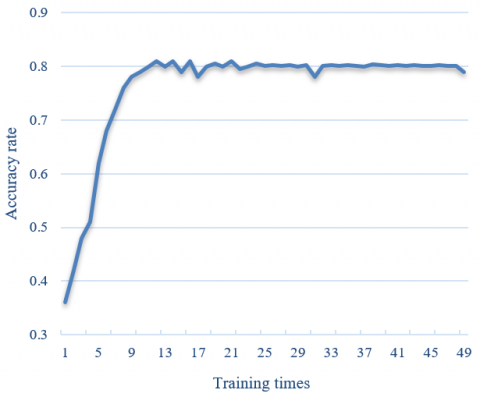(1)

## 1.png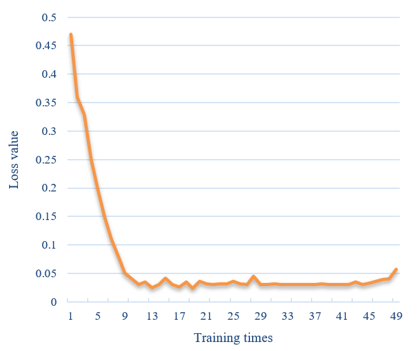(2)

Figure 6. Training accuracy rate and loss curves

From the change curve of accuracy rate in Figure 6-(1), it can be seen that the accuracy rate of the model for image target recognition tends to be stable after the 10th round of training, and reaches the highest value of 86.48% in the 45th round of training. Correspondingly, from the loss change curve in Figure 6-(2), it can be seen that the change rate of the loss function value of the model for image target recognition gradually slows down after the 10th round of training, indicating that the model has approached an overfitting state.

## 71.png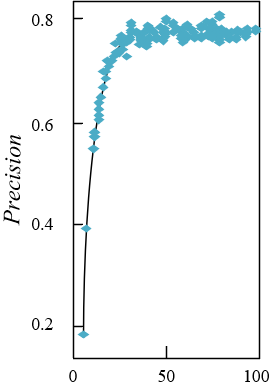(1)

## 72.png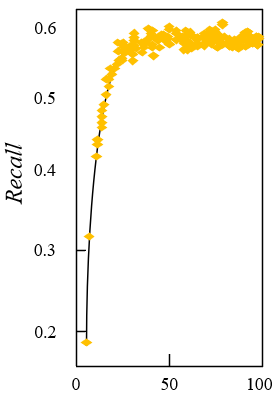(2)

## 73.png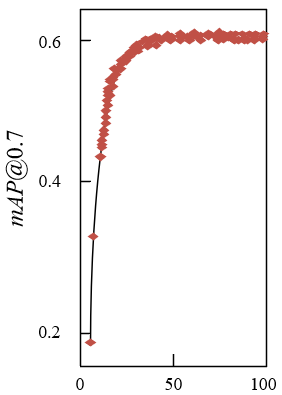(3)

## 74.png(4)

Figure 7. Experimental results of model performance

Figure 7 shows the experimental results of the performance of the model for fast recognition of image targets. It can be seen from the figure that the precision rate, recall rate and mAP values of the models under different IoU thresholds are getting higher and higher as the training time goes by.

In order to further verify the effectiveness of the proposed algorithm for image target feature extraction, a comparison of the mAP cases of different algorithms is carried out in this article, and the comparison results are given by Table 2. Figure 8 shows the mAP curves of different algorithms.

Table 2. Comparison of mAP based on different algorithms

 Models mAP% @0.7 @0.8 @0.9 Faster-RCNN 72.8 42.7 42.7 YOLOv3 71.9 55.6 49.3 Action-RCNN 72.5 59.2 47.8 YOLOv5 87.1 61.7 55.1 The improved Faster-RCNN in this article 76.9 55.8 43.6 The improvedYOLOv5 in this article 83.7 69.1 51.8

From the above table, it can be seen that the performance of the improved image target recognition model YOLOv5 in this article is slightly inferior to that of the YOLOv5-based model when the IoU threshold is set to 0.7. Due to the high cost of convolutional operations, other models fail to use the timing information of continuous image frame samples of surveillance video, so the model in this article demonstrates a superior recognition performance.

## 8.png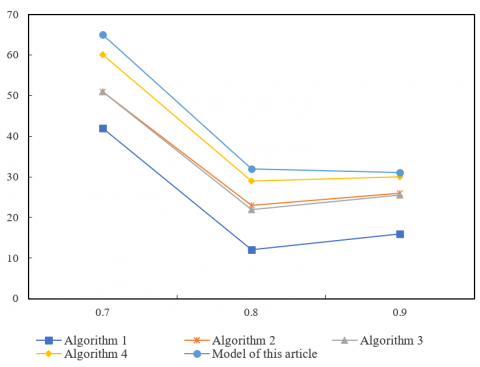Figure 8. The mAP curves of different algorithms

6. Conclusions

This article conducts studies on the fast target recognition method based on multi-scale fusion and deep learning. The optimized local binary pattern algorithm and the HOG algorithm are used to extract the image target features, the dimension reduction of the extracted image target features is carried out based on the generalized discriminant analysis algorithm, and multi-scale fusion of image targets is accomplished based on the discriminant correlation analysis. Combined with the experiment, the comparison of the performances of different image target recognition algorithms is carried out, and it is verified that the proposed improved algorithm has significantly improved target recognition accuracy rate compared with the original algorithm. Moreover, the training accuracy rate as well as the loss curves are shown, and the experimental results of the model’s performance for fast recognition of image targets are demonstrated. The results show that the precision rate, recall rate and mAP values of the models under different IoU threshold values all increase with the training time. The mAPs of different algorithms are also compared, which shows the model in this article demonstrates superior recognition performance.

Acknowledgment

This paper was funded by the High-level Talents Funding Project of Hebei Province (Grant No.: A202105006); and the Hebei Provincial Higher Education Science and Technology Research Key Project (Grant No.: ZD2021317).

References

 Fan, Y., Shen, G., Yuan, X., Xu, J. (2020). Target track recognition from few-labeled radar data with outliers. In International Conference on Urban Intelligence and Applications, Taiyuan, China, pp. 206-214. https://doi.org/10.1007/978-981-33-4601-7_21

 Lin, S., Lu, F., Shi, Y. (2020). Optimization of SAR Image Target Recognition based on Deep Learning. In 2020 13th International Conference on Intelligent Computation Technology and Automation (ICICTA), Xi'an, China, pp. 342-345. https://doi.org/10.1109/ICICTA51737.2020.00079

 Sun, X., Yin, X., Yin, Y., Liu, P., Wang, L., Tang, R. (2020). Underwater acoustic target recognition based on ReLU gated recurrent unit. In 2020 6th International Conference on Robotics and Artificial Intelligence, New York, NY, United States, pp. 41-46. https://doi.org/10.1145/3449301.3449309

 Sun, T., Wang, S., Zhang, H., Zhao, Y., Xiao, Y. (2021). Multi-target recognition method based on heatable marker. In 2021 40th Chinese Control Conference (CCC), Shanghai, China, pp. 3876-3881. https://doi.org/10.23919/CCC52363.2021.9549284

 Lv, C., Cao, L. (2021). Target recognition algorithm based on optical sensor data fusion. Journal of Sensors, 2021: Article ID 1979523. https://doi.org/10.1155/2021/1979523

 Zha, B.T., Zheng, Z., Li, H.J., Chen, G.S., Yuan, H.L. (2021). Laser fuze specific target recognition based on grey system theory. Optik, 247: 168020. https://doi.org/10.1016/j.ijleo.2021.168020

 Ning, Q., Lu, H., Shi, X., Leng, Z., Qin, P. (2021). Research on pedestrian target recognition algorithm in low light environment. In 2021 IEEE 15th International Conference on Electronic Measurement & Instruments (ICEMI), Nanjing, China, pp. 485-489. https://doi.org/10.1109/ICEMI52946.2021.9679556

 Shi, B., Zhang, Q., Li, Y., Wu, M. (2021). SAR image target recognition algorithm based on improved residual shrinkage network. In 2021 2nd International Seminar on Artificial Intelligence, Networking and Information Technology (AINIT), Shanghai, China, pp. 84-87. https://doi.org/10.1109/AINIT54228.2021.00026

 Zhang, Q., Da, L., Zhang, Y., Hu, Y. (2021). Integrated neural networks based on feature fusion for underwater target recognition. Applied Acoustics, 182: 108261. https://doi.org/10.1016/j.apacoust.2021.108261

 Liao, L., Du, L., Chen, J. (2021). Class factorized complex variational auto-encoder for HRR radar target recognition. Signal Processing, 182: 107932. https://doi.org/10.1016/j.sigpro.2020.107932

 Zhang, Q., Bao, X., Wu, B., Tu, X., Jin, Y., Luo, Y., Zhang, N. (2021). Water meter pointer reading recognition method based on target-key point detection. Flow Measurement and Instrumentation, 81: 102012. https://doi.org/10.1016/j.flowmeasinst.2021.102012

 Zhang, Y., Yuan, H., Li, H., Chen, J., Niu, M. (2021). Meta-learner-based stacking network on space target recognition for ISAR images. IEEE Journal of Selected Topics in Applied Earth Observations and Remote Sensing, 14: 12132-12148. https://doi.org/10.1109/JSTARS.2021.3128938

 Kamel, M.M., Taha, H.S., Salama, G.I., Elhalwagy, Y.Z. (2019). Ground target localization and recognition via descriptors fusion. In IOP Conference Series: Materials Science and Engineering, 610(1): 012015. https://doi.org/10.1088/1757-899X/610/1/012015

 Xu, L., Liu, G., Cao, B., Zhang, P., Liu, S. (2019). Infrared target recognition based on improved convolution neural network. In Proceedings of the 2019 8th International Conference on Networks, Communication and Computing, New York, NY, United States, pp. 83-87. https://doi.org/10.1145/3375998.3376000

 Wang, L., Wen, C., Wu, L. (2019). Target identity recognition method based on trusted information fusion. International Journal of Performability Engineering, 15(4): 1235-1246. https://doi.org/10.23940/ijpe.19.04.p19.12351246

 Wan, J., Chen, B., Xu, B., Liu, H., Jin, L. (2019). Convolutional neural networks for radar HRRP target recognition and rejection. EURASIP Journal on Advances in Signal Processing, 2019(1): 1-17. https://doi.org/10.1186/s13634-019-0603-y

 Wang, J., Liu, C., Fu, T., Zheng, L. (2019). Research on automatic target detection and recognition based on deep learning. Journal of Visual Communication and Image Representation, 60: 44-50. https://doi.org/10.1016/j.jvcir.2019.01.017

 Bai, Z. (2019). Airport target recognition and ground detection management based on neural network. International Journal of Mechatronics and Applied Mechanics, 2019(6): 190-195.

 Du, C., Chen, B., Xu, B., Guo, D., Liu, H. (2019). Factorized discriminative conditional variational auto-encoder for radar HRRP target recognition. Signal Processing, 158: 176-189. https://doi.org/10.1016/j.sigpro.2019.01.006

 Tian, Y., Duan, H., Luo, R., Zhang, Y., Jia, W., Lian, J., Li, C. (2019). Fast recognition and location of target fruit based on depth information. IEEE Access, 7: 170553-170563.

 Wang, K., Zhang, G., Leung, H. (2019). SAR target recognition based on cross-domain and cross-task transfer learning. IEEE Access, 7: 153391-153399. https://doi.org/10.1109/ACCESS.2019.2955566

 Sun, H. (2022). Image target detection and recognition method using deep learning. Advances in Multimedia, 2022: Article ID 4751196. https://doi.org/10.1155/2022/4751196

 Liu, Q.P., Wang, Q.J., Hanajima, N., Su, B. (2022). An improved method for small target recognition based on faster RCNN. In Proceedings of 2021 Chinese Intelligent Systems Conference 804: 305-313. https://doi.org/10.1007/978-981-16-6324-6_32

 Tang, Y., Chen, J. (2022). A multi-view SAR target recognition method using feature fusion and joint classification. Remote Sensing Letters, 13(6): 631-642. https://doi.org/10.1080/2150704X.2022.2063038

 He, C., Li, D., Wang, S. (2020). A lightweight convolutional neural network model for target recognition. In Journal of Physics: Conference Series 1651(1): 012138. https://doi.org/10.1088/1742-6596/1651/1/012138

 Gu, C., Dong, Z. (2020). Automatic target recognition for CT-based airport screening system. In Journal of Physics: Conference Series, 1453(1): 012111. https://doi.org/10.1088/1742-6596/1453/1/012111